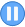﻿

## GMAT Practice Question Set #20 (Question 58-60)

Data Sufficiency Question #58: Remainders When Dividing
 Time 0 0 : 0 0 : 0 0Given two positive integers A and B such that A > B, what is the remainder when the square of B is subtracted from the square of A and then divided by 15?   (1) When the sum of A and B is divided by 5, the remainder is 1   (2) When B is subtracted from A and then divided by 3, the remainder is 1. (A)Statement 1 alone is sufficient but statement 2 alone is not sufficient to answer the question asked. (B)Statement 2 alone is sufficient but statement 1 alone is not sufficient to answer the question asked. (C)Both statements 1 and 2 together are sufficient to answer the question but neither statement is sufficient alone. (D)Each statement alone is sufficient to answer the question. (E)Statements 1 and 2 are not sufficient to answer the question asked and additional data is needed to answer the statements.GMATTM is a registered trademark of the Graduate Management Admission CouncilTM. The Graduate Management Admission CouncilTM does not endorse, nor is it affiliated in any way with the owner or any content of this web site.